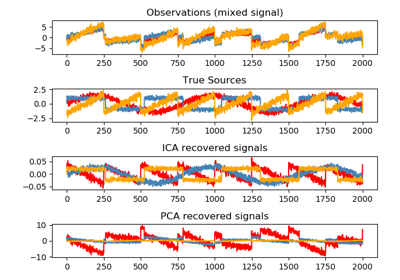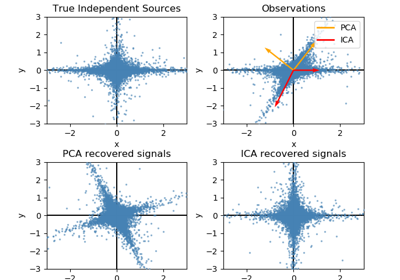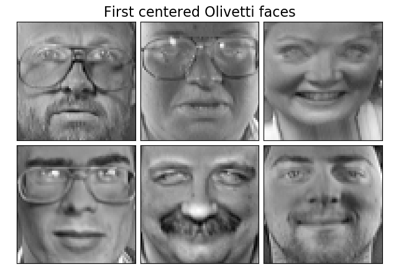# sklearn.decomposition.FastICA¶

class sklearn.decomposition.FastICA(n_components=None, algorithm='parallel', whiten=True, fun='logcosh', fun_args=None, max_iter=200, tol=0.0001, w_init=None, random_state=None)[source]

FastICA: a fast algorithm for Independent Component Analysis.

Read more in the User Guide.

Parameters
n_componentsint, optional

Number of components to use. If none is passed, all are used.

algorithm{‘parallel’, ‘deflation’}

Apply parallel or deflational algorithm for FastICA.

whitenboolean, optional

If whiten is false, the data is already considered to be whitened, and no whitening is performed.

funstring or function, optional. Default: ‘logcosh’

The functional form of the G function used in the approximation to neg-entropy. Could be either ‘logcosh’, ‘exp’, or ‘cube’. You can also provide your own function. It should return a tuple containing the value of the function, and of its derivative, in the point. Example:

def my_g(x):

return x ** 3, (3 * x ** 2).mean(axis=-1)

fun_argsdictionary, optional

Arguments to send to the functional form. If empty and if fun=’logcosh’, fun_args will take value {‘alpha’ : 1.0}.

max_iterint, optional

Maximum number of iterations during fit.

tolfloat, optional

Tolerance on update at each iteration.

w_initNone of an (n_components, n_components) ndarray

The mixing matrix to be used to initialize the algorithm.

random_stateint, RandomState instance or None, optional (default=None)

If int, random_state is the seed used by the random number generator; If RandomState instance, random_state is the random number generator; If None, the random number generator is the RandomState instance used by np.random.

Attributes
components_2D array, shape (n_components, n_features)

The linear operator to apply to the data to get the independent sources. This is equal to the unmixing matrix when whiten is False, and equal to np.dot(unmixing_matrix, self.whitening_) when whiten is True.

mixing_array, shape (n_features, n_components)

The pseudo-inverse of components_. It is the linear operator that maps independent sources to the data.

mean_array, shape(n_features)

The mean over features. Only set if self.whiten is True.

n_iter_int

If the algorithm is “deflation”, n_iter is the maximum number of iterations run across all components. Else they are just the number of iterations taken to converge.

whitening_array, shape (n_components, n_features)

Only set if whiten is ‘True’. This is the pre-whitening matrix that projects data onto the first n_components principal components.

Notes

Implementation based on A. Hyvarinen and E. Oja, Independent Component Analysis: Algorithms and Applications, Neural Networks, 13(4-5), 2000, pp. 411-430

Examples

>>> from sklearn.datasets import load_digits
>>> from sklearn.decomposition import FastICA
>>> transformer = FastICA(n_components=7,
...         random_state=0)
>>> X_transformed = transformer.fit_transform(X)
>>> X_transformed.shape
(1797, 7)


Methods

 fit(self, X[, y]) Fit the model to X. fit_transform(self, X[, y]) Fit the model and recover the sources from X. get_params(self[, deep]) Get parameters for this estimator. inverse_transform(self, X[, copy]) Transform the sources back to the mixed data (apply mixing matrix). set_params(self, \*\*params) Set the parameters of this estimator. transform(self, X[, copy]) Recover the sources from X (apply the unmixing matrix).
__init__(self, n_components=None, algorithm='parallel', whiten=True, fun='logcosh', fun_args=None, max_iter=200, tol=0.0001, w_init=None, random_state=None)[source]

Initialize self. See help(type(self)) for accurate signature.

fit(self, X, y=None)[source]

Fit the model to X.

Parameters
Xarray-like, shape (n_samples, n_features)

Training data, where n_samples is the number of samples and n_features is the number of features.

yIgnored
Returns
self
fit_transform(self, X, y=None)[source]

Fit the model and recover the sources from X.

Parameters
Xarray-like, shape (n_samples, n_features)

Training data, where n_samples is the number of samples and n_features is the number of features.

yIgnored
Returns
X_newarray-like, shape (n_samples, n_components)
get_params(self, deep=True)[source]

Get parameters for this estimator.

Parameters
deepbool, default=True

If True, will return the parameters for this estimator and contained subobjects that are estimators.

Returns
paramsmapping of string to any

Parameter names mapped to their values.

inverse_transform(self, X, copy=True)[source]

Transform the sources back to the mixed data (apply mixing matrix).

Parameters
Xarray-like, shape (n_samples, n_components)

Sources, where n_samples is the number of samples and n_components is the number of components.

copybool (optional)

If False, data passed to fit are overwritten. Defaults to True.

Returns
X_newarray-like, shape (n_samples, n_features)
set_params(self, **params)[source]

Set the parameters of this estimator.

The method works on simple estimators as well as on nested objects (such as pipelines). The latter have parameters of the form <component>__<parameter> so that it’s possible to update each component of a nested object.

Parameters
**paramsdict

Estimator parameters.

Returns
selfobject

Estimator instance.

transform(self, X, copy=True)[source]

Recover the sources from X (apply the unmixing matrix).

Parameters
Xarray-like, shape (n_samples, n_features)

Data to transform, where n_samples is the number of samples and n_features is the number of features.

copybool (optional)

If False, data passed to fit are overwritten. Defaults to True.

Returns
X_newarray-like, shape (n_samples, n_components)

## Examples using sklearn.decomposition.FastICA¶